# What Is A Geometric Series? (10 Common Questions Answered)

Geometric series are used in mathematics whenever we want to add up a sequence of numbers that grows or shrinks by a fixed percentage at each step.  However, there are a few things you should know about these series.

So, what is a geometric series?  A geometric series is a sum of a sequence of numbers that increases or decreases by the same percentage at each step. The common ratio r between consecutive terms in a geometric sequence is always the same. The sum of a geometric series with first term a is given by a / (1 – r) for |r| < 1.

Of course, a geometric sequence can have positive terms, negative terms, or both.  The common ratio r can also be positive or negative, and the first term a can be positive or negative.

Let’s get started.

## What Is A Geometric Series?

A geometric series is the sum of a geometric sequence (an infinite sequence of numbers that increases or decreases by the same percentage at each step).  The ratio r between two consecutive terms in a geometric sequence is always the same.

### How To Find The Sum Of A Geometric Series

The first term of a geometric sequence is given by a.  If we add up all of the infinitely many terms in a geometric sequence, we get:

• a + ar + ar2 + ar3 + … =  a / (1 – r)

as long as |r| < 1 (that is, -1 < r < 1).  If |r| >= 1, then the sum of the series does not converge to a finite value.

To prove that the formula above works, we will start by multiplying the left side by the denominator of the right side:

• (a + ar + ar2 + ar3 + …)(1 – r)
• =a(1 + r + r2 + r3 + …)(1 – r)  [factor out a from the first parentheses, since it appears in every term]
• =a[1(1 – r) + r(1 – r) + r2(1 – r) + r3(1 – r) + …]  [distribute the (1 – r) to every term in the series]
• =a[(1 – r) + (r – r2) + (r2 – r3) + (r3 – r4) + …]
• =a[1 – r + r – r2 + r2 – r3 + r3 – r4 + …]  [ungroup the pairs of terms]
• =a[1 – rn[as n goes to infinity]
• =a[1 – 0]  [if |r| < 1, then rn goes to zero as n goes to infinity]
• =a

Since (a + ar + ar2 + ar3 + …)(1 – r) = a, we can divide both sides by 1 – r (which is nonzero) to get the formula we wrote earlier:

• a + ar + ar2 + ar3 + … =  a / (1 – r)

### How To Find The Partial Sum Of A Geometric Series (Adding Up A Finite Number Of Terms)

To find the partial sum of a geometric series (the first n terms, starting with a and going to arn-1), we use the formula:

• a + ar + ar2 + ar3 + … arn-1 =  a(1 – rn) / (1 – r)

for |r| < 1 (or -1 < r < 1).

In the case of r = 1, we add up the value of a, n times in a row, giving us a sum of na.

For the case r = -1, we will get a sum of zero if n is even and a sum of a if n is odd.

## Do All Geometric Series Converge?

Not all geometric series converge.  Any geometric series with |r| >= 1 will diverge.

The reason is that we are adding up infinitely many terms that are increasing in absolute value.  So, the sum gets further away from zero as we continue to add more terms.

Since there is no upper boundary to the absolute value of the sum, it does not converge to a finite value.

### Example: A Geometric Series That Does Not Converge

Consider the geometric series with a = 1 and r = 2.  The series looks like:

• 1 + 2 + 4 + 8 + 16 + 32 + 64 + …

Every term is positive and larger than the term before it.  Since the terms increase without bound, the sum will increase every time we add another term.

Since |r| = |2| = 2 > 1, the sum of the series will not converge to a finite value.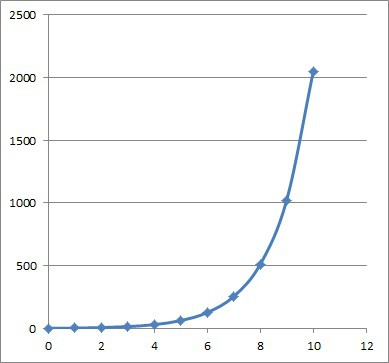This is the graph of the first 10 partial sums of the geometric series with a = 1 and r =2. The value of the sums will continue to grow.

## Can A Geometric Series Be Negative?

A geometric series can be negative.  It could have all of its terms negative (a < 0 and r > 0) or half of its terms negative (r < 0).

### Can A Geometric Series Be Alternating?

A geometric series can be alternating (that is, its terms can oscillate between positive and negative values).  This can happen if the value of r is negative (that is, r < 0).

If -1 < r < 0, the series converges, but if r <= -1, the series diverges.

#### Example 1: An Alternating Geometric Series That Converges

Consider the geometric series with a = 1 and r = -1/2.  The series looks like:

• 1 – 1/2 + 1/4 – 1/8 + 1/16 – 1/32 + 1/64 – …

Every term with an even index is positive, and every term with an odd index is positive, which means we have an alternating series.

Since |r| = |-1/2| = 1/2 < 1, we can still use the formula for the sum of a geometric series:

• a / (1 – r)

Substituting a = 1 and r = -1/2, we get:

• 1 / (1 – (-1/2))
• =1 / (1 + (1/2))
• =1 / (3/2)
• =2/3

So the sum of this geometric series is 2/3 (a finite value), meaning it converges.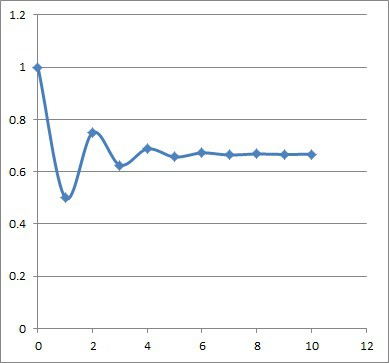This is the graph of the first 10 partial sums of the geometric series with a = 1 and r =-1/2. The value of the sums will converge to a value of 2/3.

#### Example 2: An Alternating Geometric Series That Diverges

Consider the geometric series with a = 1 and r = -2.  The series looks like:

• 1 – 2 + 4 – 8 + 16 – 32 + 64 – …

Every term with an even index is positive, and every term with an odd index is positive, which means we have an alternating series.

Since |r| = |-2| = 2 > 1, we cannot use the formula for the sum of a geometric series.  In this case, the series diverges (does not converge to a finite sum).

We can see this with the graph of the first few partial sums below.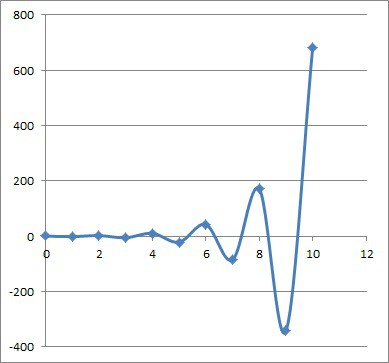This is the graph of the first 10 partial sums of the geometric series with a = 1 and r = -2. The absolute value of the sums will continue to grow, although their signs will continue to alternate.

### Can The Sum Of A Geometric Series Be Negative?

The sum of a geometric series can be negative in two ways:

• If all of the terms are negative (a < 0 and r > 0), then the sum will be negative (we still need |r| < 1 for the series to converge).
• If the series is alternating with negative first term (a < 0 and r < 0), the sum will be negative (we still need |r| < 1 for the series to converge).

#### Example 1: A Geometric Series With A Negative Sum (a < 0 & r > 0)

Consider the geometric series with a = -1 and r = 1/2.  The series looks like:

• -1 – 1/2 – 1/4 – 1/8 – 1/16 – 1/32 – 1/64 – …

Every term of the series is negative.  Since |r| = |1/2| = 1/2 < 1, we can still use the formula for the sum of a geometric series:

• a / (1 – r)

Substituting a = -1 and r = 1/2, we get:

• -1 / (1 – (1/2))
• =-1 / (1/2)
• =-2

So the sum of this geometric series is -2 (a finite value), meaning it converges.

Note that the first term has a value of -1 (half of the sum), and the rest of the terms sum to -1 (the other half of the sum).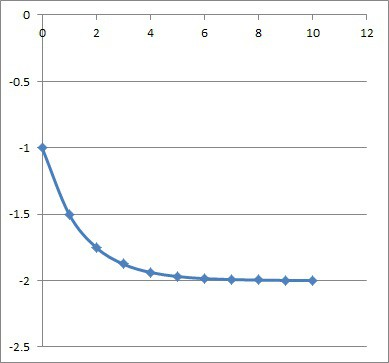This is the graph of the first 10 partial sums of the geometric series with a = -1 and r =1/2. The value of the sums will converge to a value of -2.

#### Example 2: A Geometric Series With A Negative Sum (a < 0 & r < 0)

Consider the geometric series with a = -1 and r = -1/2.  The series looks like:

• -1 + 1/2 – 1/4 + 1/8 – 1/16 + 1/32 – 1/64 + …

The terms are alternating, with even index terms being negative and odd index terms being positive.

Since |r| = |-1/2| = 1/2 < 1, we can still use the formula for the sum of a geometric series:

• a / (1 – r)

Substituting a = -1 and r = -1/2, we get:

• -1 / (1 – (-1/2))
• -1 / (1 + (1/2))
• =-1 / (3/2)
• =-2/3

So the sum of this geometric series is -2/3 (a finite value), meaning it converges.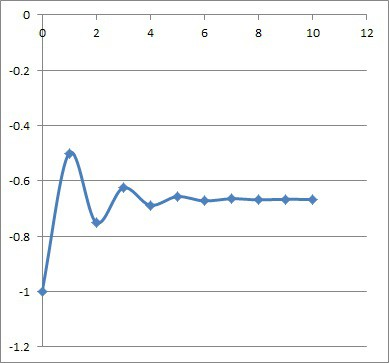This is the graph of the first 10 partial sums of the geometric series with a = -1 and r =-1/2. The value of the sums will converge to a value of -2/3.

## Is An Annuity A Geometric Series?

An annuity is an example of a geometric series with an initial payout (the value of a) and a percentage change in the value of the payout every year (the value of r).

### Example: An Annuity As A Geometric Series

Let’s say that you are to receive an annuity starting this year.  The payments will be \$50,000 per year.

However, with inflation of 4%, the true value of the annuity payment will decrease every year (that is, the purchasing power of your annuity will decline by 4% from the previous year).

So every year, the true value of the payment will decrease by 4% (that is, we multiply the true value of this year’s payment by a factor of 0.96 to get the present value of next year’s payment).

So, the present value of the annuity payments will be:

50,000 + 50,000(0.96) + 50,000(0.96)2 + 50,000(0.96)3 + …

This is a geometric series with a = 50,000 and r = 0.96.  Since |r| = |0.96| = 0.96 < 1, we can use the formula for the sum of a geometric series:

• a / (1 – r)
• =50,000 / (1 – 0.96)
• =50,000 / (0.04)
• =1,250,000

So, your annuity payments have a present value of \$1.25 million dollars.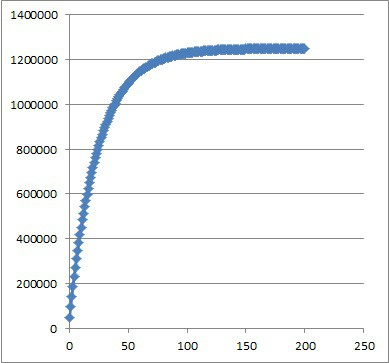An annuity with an annual payment of \$50,000 has a limit on its present value, based on inflation.

## Is A Geometric Series An Exponential Function?

A geometric series is not an exponential function.

However, a geometric series is the sum of the terms of a geometric sequence.  Those terms have values that are an exponential function f(x) = arx evaluated at values of x when x is a natural number (x = 0, 1, 2, 3, …).

You can see the graph of the terms of a geometric sequence below.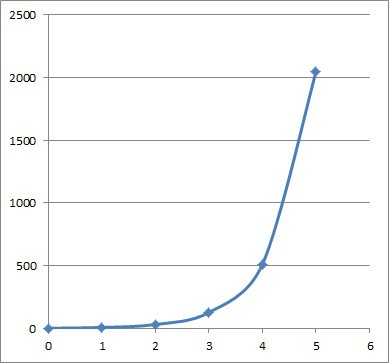The first few terms of the geometric sequence with first term a = 4 and common ratio r = 4.

## Conclusion

Now you know what geometric series are and what they look like.  You also know the answers to some common questions about geometric series.